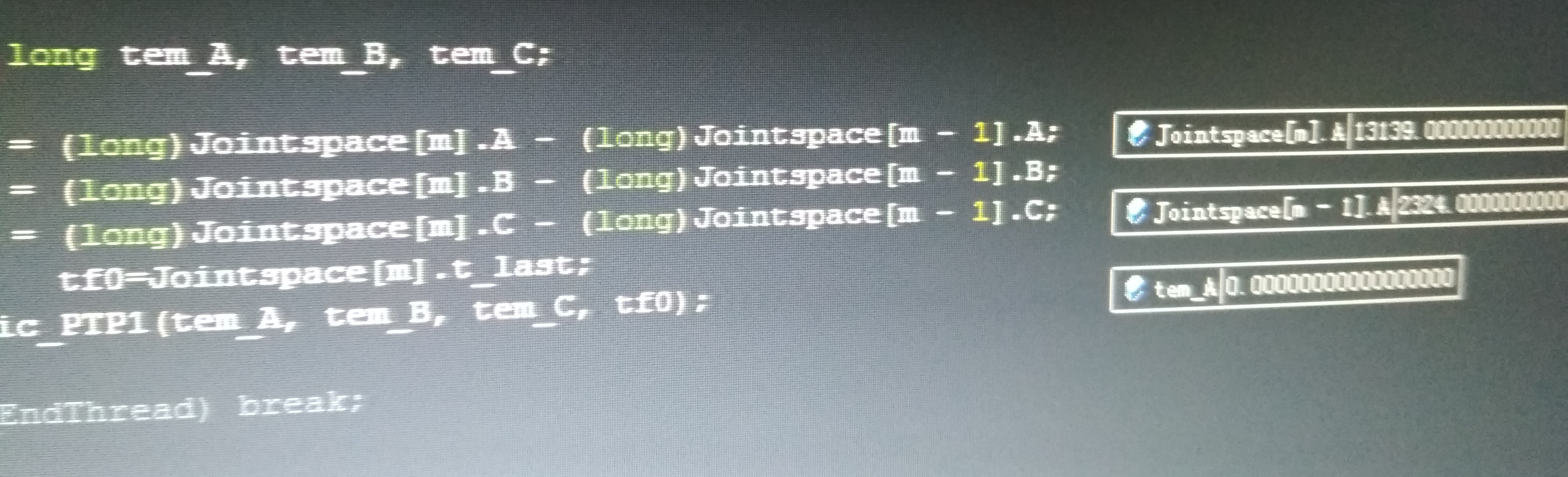# MFC,线程调用函数

.h头文件声明 自定义函数：void Denmic（long a long b);

UINT timef （LPVOID aParm）
{
return 0;
}

...全文
1953 点赞 收藏 236

236 条回复

schlafenhamster 2018-07-07
for(short i=0;i<p_inx; i++)//这里的i可以累加了
{
if(i==0) 这条语句 只含 下一句
afxDump<<i<<"=i\n"; // if 到这里 结束 ！！！
afxDump<<p_inx<<"=p_inx\n";//是这样改的
{ // 这个 大 括号 与 if （i==0）无关 ！！！！
long a=Jspace[i].a;
******
}

qq_37834095 2018-07-07

qq_37834095 2018-07-03

qq_37834095 2018-07-03
UNIT timmef1(LPVOID aparm)
{

for(short i=0;i<p_inx; i++)//这里的i可以累加了
{
if(i==0)
afxDump<<i<<"=i\n";
afxDump<<p_inx<<"=p_inx\n";
//是这样改的
{
long a=Jspace[i].a;
******
}
else
{

long tm_a,tm_b,tm_c;
}

qq_37834095 2018-07-03schlafenhamster 2018-07-03
if(i==0)
afxDump<<i<<"=i\n";// if end !!!!

afxDump<<p_inx<<"=p_inx\n";
{

afxDump<<i<<"=i\n";
afxDump<<p_inx<<"=p_inx\n";
if(i==0)
{

qq_37834095 2018-07-03

qq_37834095 2018-07-03
for(short i=0;i<Pinx;i++)// 共 pinx 个 线程 ！！！
{
if(i==0)
{
}
else
{
}
}

UNIT timmef1(LPVOID aparm)
{

long tm_a,tm_b,tm_c;
for(short i=0;i<p_inx; i++)//这里的i可以累加了
{
if(i==0)
afxDump<<i<<"=i\n";
afxDump<<p_inx<<"=p_inx\n";
{
long a=Jspace[i].a;
******
*****

Denmic( a ,b,c,tf0);
}
else
{
tm_a=(long)Jspace[i].a-(long)Jspace[i- 1].a;//这里2个变量值做减法，明明有值的，为什么tm_a=0呢？
tm_b=(long)Jspace[i].a-(long)Jspace[i- 1].b;
tm_c=(long)Jspace[i].a-(long)Jspace[i- 1].c;

Denmic(tm_a,tm_b,tm_c,(long)Jspace[i].c,(long)Jspace[i].l_time);
}

}

return 0;

}

schlafenhamster 2018-07-03
for(short i=0;i<Pinx;i++)// 共 pinx 个 线程 ！！！
{
if(i==0)
{
}
else
{
}
}

qq_37834095 2018-07-03

UNIT timmef1(LPVOID aparm)
{

long tm_a,tm_b,tm_c;
for(short i=0;i<p_inx; i++)//这里的i不累加是线程调用的Denmic函数在死循环…………
{
if(i==0)
afxDump<<i<<"=i\n";
afxDump<<p_inx<<"=p_inx\n";
{

Denmic( (long)Jspace[i].a ,(long)Jspace[i].b,(long)Jspace[i].c,(long)Jspace[i].l_time);
}
else
{
tm_a=(long)Jspace[i].a-(long)Jspace[i- 1].a;
tm_b=(long)Jspace[i].a-(long)Jspace[i- 1].b;
tm_c=(long)Jspace[i].a-(long)Jspace[i- 1].c;

Denmic(tm_a,tm_b,tm_c,(long)Jspace[i].c,(long)Jspace[i].l_time);
}

}

return 0;

}

schlafenhamster 2018-07-02
"就一个线程啊"
if(short i=0;i<Pinx;i++)

qq_37834095 2018-07-01

void xxDlg::OnButtonStart()//启动按钮

{

if(short i=0;i<Pinx;i++)//这里的i是全局的
{
if(i==0)
{
}
else
{
}
}

}

qq_37834095 2018-07-01

UNIT timmef1(LPVOID aparm)
{

long tm_a,tm_b,tm_c;
for(short i=0;i<p_inx; i++)//这里的i不累加是线程调用的Denmic函数在死循环…………
{
if(i==0)
afxDump<<i<<"=i\n";
afxDump<<p_inx<<"=p_inx\n";
{

Denmic( (long)Jspace[i].a ,(long)Jspace[i].b,(long)Jspace[i].c,(long)Jspace[i].l_time);
}
else
{
tm_a=(long)Jspace[i].a-(long)Jspace[i- 1].a;
tm_b=(long)Jspace[i].a-(long)Jspace[i- 1].b;
tm_c=(long)Jspace[i].a-(long)Jspace[i- 1].c;

Denmic(tm_a,tm_b,tm_c,(long)Jspace[i].c,(long)Jspace[i].l_time);
}

}

return 0;

}

schlafenhamster 2018-07-01

else
{
}

schlafenhamster 2018-06-29

UNIT timmef1(LPVOID aparm)
{
{
。。。。。。。。。。。。。。。。。。
}

i =0 时 Denmic （） 因为 while 而在 Denmic（） 内 死循环， 当然 i没法 i++ ！

qq_37834095 2018-06-29
UNIT timmef1(LPVOID aparm)
{

long tm_a,tm_b,tm_c;
for(short i=0;i<p_inx; i++)//这里的i为什么不循环累加呢？
{
if(i==0)
afxDump<<i<<"=i\n";
afxDump<<p_inx<<"=p_inx\n";
{

Denmic( (long)Jspace[i].a ,(long)Jspace[i].b,(long)Jspace[i].c,(long)Jspace[i].l_time);
}
else
{
tm_a=(long)Jspace[i].a-(long)Jspace[i- 1].a;
tm_b=(long)Jspace[i].a-(long)Jspace[i- 1].b;
tm_c=(long)Jspace[i].a-(long)Jspace[i- 1].c;

Denmic(tm_a,tm_b,tm_c,(long)Jspace[i].c,(long)Jspace[i].l_time);
}

}

return 0;

}

//----------------------------------------------------------------------
Denmic(long a,long b,long c,long t0)
{

long count =0;

double os;tim=0,t0;
double rfos_x,rfos_y,rfos_z;
short space_x,space_y,space_z;

{

if (space_x>0&&space_y>0&&space_z>0)
{
if(time<t0)
{
T+=6;

os=a*T*T/(t0*t0);
os=b*T*T/(t0*t0);
os=c*T*T/(t0*t0);

for(short i=1;i<=3;i++)
{
te(i,os[3-i]);

}

}

if(time==t0)

{
Stop(a,tf);
Stop(b,tf);
Stop(c,tf);
}
}

else if ((space_x==0)&&(space_y==0)&&(space_z==0))

{
Start(x);
Start(y);
Start(z);
}
count++;

}

}

void xxDlg::OnButtonStart()//启动按钮

{

}

schlafenhamster 2018-06-29

1.5w+

VC/MFC 进程/线程/DLL